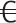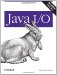# Decimal Formats

The java.text.DecimalFormat class provides even more control over how floating-point numbers are formatted:

```public class DecimalFormat extends NumberFormat
```

Most number formats are in fact decimal formats. Generally, you can simply cast any number format to a decimal format, like this:

```DecimalFormat df = (DecimalFormat) NumberFormat.getCurrencyInstance( );
```

At least in theory, you might encounter a nondecimal format. Therefore, you should use instanceof to test whether or not youve got a DecimalFormat:

```NumberFormat nf = NumberFormat.getCurrencyInstance( );
if (nf instanceof DecimalFormat) {
DecimalFormat df = (DecimalFormat) NumberFormat.getCurrencyInstance( );
//...
}
```

Alternatively, you can place the cast and associated operations in a TRy/catch block that catches ClassCastExceptions:

```try {
DecimalFormat df = (DecimalFormat) NumberFormat.getCurrencyInstance( );
//...
}
catch (ClassCastException ex) {System.err.println(ex);}
```

#### .6.1. Decimal Format Patterns

Every DecimalFormat object has a pattern that describes how numbers are formatted and a list of symbols that describes with which characters they e formatted. This allows the single DecimalFormat class to be parameterized so that it can handle many different formats for different kinds of numbers in many locales. The pattern is given as an ASCII string. The symbols are provided by a DecimalFormatSymbols object. These are accessed and manipulated through the following six methods:

```public DecimalFormatSymbols getDecimalFormatSymbols( )
public void setDecimalFormatSymbols(DecimalFormatSymbols newSymbols)
public String toPattern( )
public String toLocalizedPattern( )
public void applyPattern(String pattern)
public void applyLocalizedPattern(String pattern)
```

The decimal format symbols specify the characters or strings used for the zero digit, the grouping separator, the decimal sign, the percent sign, the mille percent sign, infinity (IEEE 754 Inf), not a number (IEEE 754 NaN), and the minus sign. In American English these are 0, ,, ., %,, Inf, NaN, and -, respectively. They may be other things in different locales.

The pattern specifies whether leading and trailing zeros are printed, whether the fractional part of the number is printed, the number of digits in a group (three in American English), and the leading and trailing suffixes for negative and positive numbers.

For instance, #,##0.### is the decimal format pattern for U.S. English and most other non-Arabic-speaking locales. The notable exceptions are the Arabic-speaking countries and Macedonia. The primary difference between locales comes in the decimal format symbols, not the pattern. The currency formats have more variation because most countries have their own currencies with their own unique symbols.

The # mark means any digit character except a leading or trailing zero. The comma is the grouping separator, the period is the decimal point separator, and the 0 is a digit that will be printed even if its a nonsignificant zero. Interpret this pattern as follows:

1. The integer part contains as many digits as necessary.

2. These are separated every three digits with the grouping separator.

3. If the integer part is zero, there is a single zero before the decimal separator.

4. Up to three digits are printed after the decimal separator. However, they are not printed if they are trailing zeros.

5. No separate pattern is included for negative numbers. Therefore, they will be printed the same as a positive number but prefixed with a minus sign.

You can apply your own patterns to support different formats. For example, the pattern 0.00000000E0 specifies a number will be formatted in exponential notation with exactly one digit before the decimal separator and exactly eight digits after the decimal separator.

Its relatively painful to work with this grammar directly. Fortunately, there are methods that allow you to get and set the values of these individual pieces directly, and I recommend that you use them:

```public String getPositivePrefix( )
public void setPositivePrefix(String newValue)
public String getPositiveSuffix( )
public void setPositiveSuffix(String newValue)
public String getNegativePrefix( )
public void setNegativePrefix(String newValue)
public String getNegativeSuffix( )
public void setNegativeSuffix(String newValue)
public int getMultiplier( )
public void setMultiplier(int newValue)
public int getGroupingSize( )
public void setGroupingSize(int newValue)
public boolean isDecimalSeparatorAlwaysShown( )
public void setDecimalSeparatorAlwaysShown(boolean newValue)
```

The positive prefix is the string prefixed to positive numbers. Most of the time, this is the empty string, but in some circumstances you might want to use a plus sign (+). In currency formats, the positive prefix is often set to the currency sign, like \$ or £, depending on the locale. You can also set a positive suffix; that is, a string that is appended to all positive numbers. This is used to attach the percent sign to percentage formats. The negative prefix is the minus sign (-). However, in accounting and other financial applications it may be an open parenthesis instead. In these applications, theres also a negative suffix, generally a closing parenthesis. Thus, -12 might be formatted as (12).

The multiplier is an integer by which the number is multiplied before being formatted. This is commonly used in percent formats. This allows a number like 0.85 to be formatted as 85% instead of 0.85%. 1, 100, and 1000 are the only common values of this number. Grouping size is the number of digits between grouping separators, commas in English. This is how 75365 becomes 75,365. Most locales, including English, break every three digits; a few break every four, formatting 75365 as 7,5365. Finally, you can specify whether or not the decimal separator (decimal point) is shown in numbers without fractional parts. By default, a number like 1999 does not have a decimal point. However, there are situations (C source code, for example) where the difference between 1999 and 1999. is significant.

You also have access to the following methods, inherited from java.text.NumberFormat, which allow you to set and get the minimum and maximum number of integer and fraction digits and control whether or not grouping is used at all. These work just as well with decimal formats as they do with regular number formats:

```public boolean isGroupingUsed( )
public void setGroupingUsed(boolean useGrouping)
public int getMaximumIntegerDigits( )
public void setMaximumIntegerDigits(int maxDigits)
public int getMinimumIntegerDigits( )
public void setMinimumIntegerDigits(int minDigits)
public int getMaximumFractionDigits( )
public void setMaximumFractionDigits(int maxDigits)
public int getMinimumFractionDigits( )
public void setMinimumFractionDigits(int minDigits)
```

#### .6.2. DecimalFormatSymbols

Each DecimalFormat object has a DecimalFormatSymbols object that contains a list of the different symbols used by decimal number formats in a particular locale. The decimal format symbols specify the characters or strings used for the zero digit, the grouping separator, the decimal sign, the percent sign, the mille percent sign, infinity (IEEE 754 Inf), not a number (IEEE 754 NaN), and the minus sign. DecimalFormatSymbols has two constructors, but they e rarely used:

```public DecimalFormatSymbols( )
public DecimalFormatSymbols(Locale locale)
```

```public DecimalFormatSymbols getDecimalFormatSymbols( )
```

If you create your own DecimalFormatSymbols object, perhaps for a locale Java doesn support, you can make a DecimalFormat use it by passing it to DecimalFormats setDecimalFormatSymbols( ) method:

```public void setDecimalFormatSymbols(DecimalFormatSymbols newSymbols)
```

The DecimalFormatSymbols class contains mostly getter and setter methods for inspecting and setting the values of the different symbols:

```public char getZeroDigit( )
public void setZeroDigit(char zeroDigit)
public char getGroupingSeparator( )
public void setGroupingSeparator(char groupingSeparator)
public char getDecimalSeparator( )
public void setDecimalSeparator(char decimalSeparator)
public char getMonetaryDecimalSeparator( )
public void setMonetaryDecimalSeparator(char decimalSeparator)
public char getPercent( )
public void setPercent(char percent)
public char getPerMill( )
public void setPerMill(char perMill)
public String getInfinity( )
public void setInfinity(String infinity)
public String getNaN( )
public void setNaN(String NaN)
public char getMinusSign( )
public void setMinusSign(char minusSign)
```

The zero digit is the character used for zero. This is 0 in most Western languages but is different in Arabic and a few other locales. The grouping separator is the character used to split groups; a comma is used in the U.S., but a period is used in some other countries that use a comma as the decimal separator. The decimal separator is a decimal point (a period) in English but a comma in some other locales. Most of the time regular numbers and money numbers use the same decimal separator, but in a few cases they e different. (Pre-Euro Portugal is one case.) The monetary separator specifies the decimal separator for currency formats. The percent and per mille characters are % and ? in English, occasionally other things in other locales. The infinity and not-a-number strings are rarely changed. They e Inf and NaN as specified by IEEE 754, generally even in non-English languages like German, where the word for infinity is Unbegrenztheit and "not a number" translates as "nicht eine Zahl." Finally, the minus sign is the default character used for negative numbers that do not have a specific prefix. Its a hyphen (-) in English. This character is not used if the associated pattern has set a negative prefix.

In Java 1.2 and later, there are methods to get and set the currency symbol and code:

```public String getCurrencySymbol( )
public void setCurrencySymbol(String symbol)
public String getInternationalCurrencySymbol( )
public void setInternationalCurrencySymbol(String code)
public Currency getCurrency( ) // Java 1.4
public void setCurrency(Currency currency) // Java 1.4
```

The currency symbol is the actual currency character(s) that prefix a monetary value such as \$,, or £. The currency symbol is the ISO 4217 three-letter ASCII code for that currency such as USD, EUR, or GBP. The Currency object is a Java 1.4 typesafe enum that encapsulates the available currencies. These three values should be in sync. Setting the currency or the currency symbol will also set the other two properties to corresponding values. For example, changing the international currency symbol to EUR, changes the currency symbol to. You don have to explicitly call setCurrencySymbol( ) and setCurrency( ). The reverse procedure does not work, however. Changing the currency symbol does not change the currency and the international currency symbol.

#### .6.3. Constructing Decimal Formats with Patterns and Symbols

Most of the time, you use the factory methods in NumberFormat to get DecimalFormat instances. However, there are three public DecimalFormat constructors you can use to create DecimalFormat instances directly:

```public DecimalFormat( )
public DecimalFormat(String pattern)
public DecimalFormat(String pattern, DecimalFormatSymbols symbols)
```

The no-argument constructor creates a decimal format that uses the default pattern and symbols for the default locale. The second constructor creates a decimal format that uses the specified pattern and the default symbols for the default locale. The third constructor creates a decimal format that uses the specified pattern and the specified symbols for the default locale. These are useful for special cases that aren handled by the default patterns and symbols.Java I/O ISBN: 0596527500EAN: 2147483647 Year: 2004Pages: 244 Authors: Elliotte Rusty Harold BUY ON AMAZON Flylib.com © 2008-2020. If you may any questions please contact us: flylib@qtcs.net